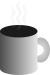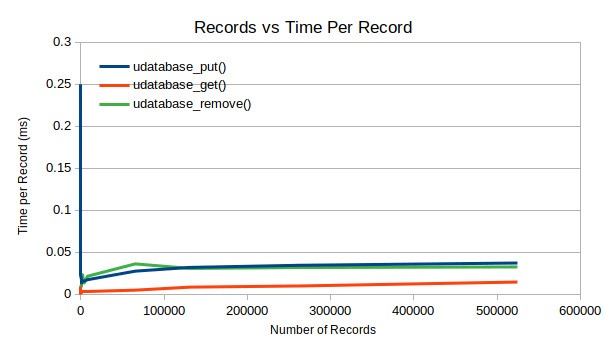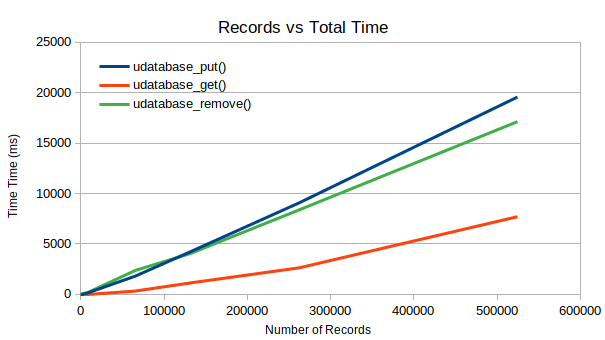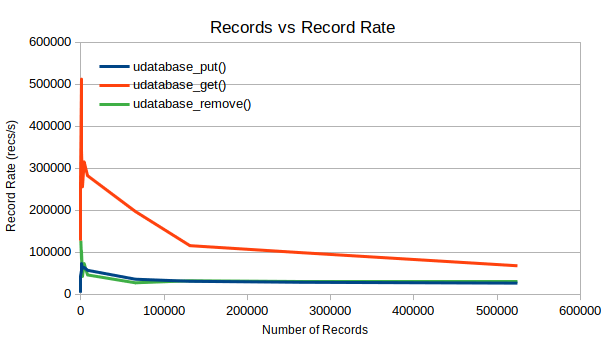#Coffee SpaceListen:

## uDatabase BugPreview Image

A little while ago I create uDatabase, an open source micro flat-file database in under 256 lines of C code.

The intention is to use uDatabase as pat of the new dead social successor. Before using it for such a project, I wanted to hack together a simple example project to put it through it's paces.

### Simple Notes

Enter simple notes, an unreleased project to write basic notes in the browser and have them saved to the database. As a result of this development, uServer got a file location bug fix and a new feature for reading form data.

The problem is, that when saving significant amounts of data, it would sometimes get concatenated. Dropping data is far from ideal when you're entire purpose as a library is to store data! Ouch!

### Current Tests

Currently, we test the code by writing random entries as follows in main.c in the function testPut():

0001 int64_t testPut(struct uDB* db, int width, int num){
0002   srand(0);
0003   char* k = malloc(32);
0004   char* test = malloc((width * 4) + num);
0005   for(int x = 0; x < (width * 4) + num; x++) test[x] = (char)rand();
0006   int64_t putStart = currentTimeMillis();
0007   for(int x = 0; x < num; x++){
0008     char* v = test + x;
0009     sprintf(k, "%x", x);
0010     int n = width * ((x % 4) + 1);
0011     if(udatabase_put(db, k, v, n) < 0){
0012       fprintf(stderr, "Failed to insert key=%s\n", k);
0013     }
0014   }
0015   return currentTimeMillis() - putStart;
0016 }

We then check that this data is read back afterwards with checkPut():

0017 int64_t checkPut(struct uDB* db, int width, int num){
0018   srand(0);
0019   char* k = malloc(32);
0020   char* test = malloc((width * 4) + num);
0021   for(int x = 0; x < (width * 4) + num; x++) test[x] = (char)rand();
0022   int64_t putStart = currentTimeMillis();
0023   for(int x = 0; x < num; x++){
0024     char* v = test + x;
0025     sprintf(k, "%x", x);
0026     int n = width * ((x % 4) + 1);
0027     char* val = udatabase_get(db, k);
0028     if(val != NULL){
0029       if(memcmp(val, v, n) != 0){
0030         fprintf(stderr, "For x=%i, key=%s, reading entry of length=%i\n", x, k, n);
0031         break;
0032       }
0033       free(val);
0034     }else{
0035       fprintf(stderr, "For x=%i, key=%s, reading entry of length=%i\n", x, k, n);
0036       fprintf(stderr, "Failed to read back value for x=%i\n", x);
0037     }
0038   }
0039   return currentTimeMillis() - putStart;
0040 }

The data to be stored, n, is calculated as $n = width \times ((x \bmod 4) + 1)$, where width is $1024-16$ (1008). We conveniently store neatly sized blocks in the database, and I believe this is where we come unstuck.

### Replication

The first task is to try and replicate this issue in our automated testing. This initially will involve having a variable length put() and get() operation. To achieve this we can change the following:

0041 int n = width * ((x % 4) + 1); // existing

To the following variable width version:

0042 int n = width + (x % (width * 3)); // new

Re-running, we get the following error:

0043 For x=1, key=1, reading entry of length=1009

This tells us that the problem starts at length 1009. This is triggered with the following code:

0044 if(memcmp(val, v, n) != 0){
0045   fprintf(stderr, "For x=%i, key=%s, reading entry of length=%i\n", x, k, n);
0046   break;
0047 }

We comment that break, re-compile, re-run and now see: We now get the following errors (which I've reduced for the purpose of readability):

0048 For x=1, key=1, reading entry of length=1009
0049 [..]
0050 For x=1007, key=3ef, reading entry of length=2015
0051 For x=1009, key=3f1, reading entry of length=2017
0052 [..]

Note that skip over exactly where our testing blocks are, sigh. It appears the problem is exactly as bad as I thought it would be.

### Digging In

My suspicion is that the problem here is insertion. udatabase_put() looks like follows:

0053 /**
0054  * udatabase_put() Store value using key. No check if the key already exists.
0055  * @param db The database description.
0056  * @param k A NULL terminated key string to be used to insert a value.
0057  * @param v A string to be inserted into the database.
0058  * @param n The length of the string to be stored.
0059  * @return The success of the insertion, 0 if success.
0060  **/
0061 int udatabase_put(struct uDB* db, char* k, char* v, long int n){
0062   char hash[UDATABASE_HLEN];
0063   udatabase_shash(k, hash, UDATABASE_HLEN);
0064   long int toff = udatabase_remove(db, k, hash); // NOTE: Remains locked.
0065   if(db != NULL && db->fp != NULL && memcmp(hash, UDATABASE_NULL, UDATABASE_HLEN) != 0){
0066     fseek(db->fp, 0, SEEK_END);
0067     if(toff >= 0){
0068       db->table[toff] = ftell(db->fp);
0069     }else{
0070       long int t = -1;
0071       while(++t < db->tableLen && db->table[toff = *(uint16_t*)hash + (UDATABASE_KLEN * t)] >= 0);
0072       if(t < db->tableLen && toff >= 0){
0073         db->table[toff] = ftell(db->fp);
0074       }else{
0075         toff = -1;
0076       }
0077     }
0078     long int x = 0;
0079     while(x < n && v != NULL){
0080       fwrite(&hash, 1, UDATABASE_HLEN, db->fp);
0081       x += fwrite(v + x, 1, (n - x) % db->dWidth != 0 ? (n - x) % db->dWidth : db->dWidth, db->fp);
0082     }
0083     while(x++ % db->dWidth != 0) fputc('\0', db->fp);
0084   }
0085   pthread_mutex_unlock(&db->lock); // NOTE: Unlock from remove() call.
0087 }

The interesting code for us is the following (with my added comments about what it is supposed to do):

0088 long int x = 0; // Offset into the data written
0089 while(x < n && v != NULL){ // Keep write out until we write everything
0090   fwrite(&hash, 1, UDATABASE_HLEN, db->fp); // Write the hash for the data
0091   /* Write the largest block we can */
0092   x += fwrite(v + x, 1, (n - x) % db->dWidth != 0 ? (n - x) % db->dWidth : db->dWidth, db->fp);
0093 }
0094 /* If we finish before the end of the, fill with zeros */
0095 while(x++ % db->dWidth != 0) fputc('\0', db->fp);

What we'll do is process these values manually. We have two cases we can test, n = 1008 (works) and n = 1009 (doesn't work). The variables we'll manually test are:

• n = 1008 and 1009.
• UDATABASE_HLEN = 16.
• db->dWidth = 1008.

For the case of 1008:

1. x = 0, n = 1008. x < n == true, continue.
2. Write 16 bytes out for the hash.
3. v + x == v, n - x == n, n = 1008. (n - x) % db->dWidth == 0, therefore write out length of db->dWidth.
4. x += db->dWidth.
5. x == db->dWidth == 1008 == n, therefore stop looping.
6. x % db->dWidth == 0, therefore end writing.

The case for 1009 is a little more complicated:

1. x = 0, n = 1009. x < n == true, continue.
2. Write 16 bytes out for the hash.
3. v + x == v, n - x == n, n = 1009. (n - x) % db->dWidth == 1, therefore write out length of (n - x) % db->dWidth == 1. Oh dear.

### Bug Fix

In the write-out line, instead of writing (n - x) % db->dWidth, we should instead be writing out as much as we possibly can to fill the block. We should change this to:

0096 x += fwrite(v + x, 1, (n - x) <= db->dWidth ? (n - x) : db->dWidth, db->fp);

And now we pass tests!

### Performance

As you can see in the following graphs, this has had little to no effect on the performance when compared to the previous results.Records vs Time Per Record

One thing worth noting is the reduced get() time. I would need to investigate this further to figure out why - but I'm not complaining.Records vs Total Time

Other than this, things look normal.Records vs Record Rate

### Conclusion

Some take-away points:

1. Testing is useful - Ideally we would all write comprehensive tests, but ultimately you will never cover all scenarios 100%. Realistically we just aim for the most likely scenarios.
2. Add failure cases to tests - When you do spot a regression not included in your current tests, and it's possible to reliably test for, you should of course add it.
3. Manual step-through is useful - Stepping through the code line by line with known good and bad data allows you to very quickly figure out how to fix it!